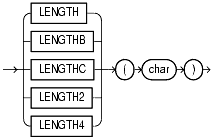## LENGTH

#### Syntax

length::=Text description of length

#### Purpose

The "length" functions return the length of `char`. `LENGTH` calculates length using characters as defined by the input character set. `LENGTHB` uses bytes instead of characters. `LENGTHC` uses Unicode complete characters. `LENGTH2` uses UCS2 codepoints. `LENGTH4` uses UCS4 codepoints.

`char` can be any of the datatypes `CHAR`, `VARCHAR2`, `NCHAR`, `NVARCHAR2`, `CLOB`, or `NCLOB`. The return value is of datatype `NUMBER`. If `char` has datatype `CHAR`, then the length includes all trailing blanks. If `char` is null, then this function returns null.

#### Examples

The following example uses the `LENGTH` function using a single-byte database character set.

```SELECT LENGTH('CANDIDE') "Length in characters"
FROM DUAL;

Length in characters
--------------------
7

```

This example assumes a double-byte database character set.

```SELECT LENGTHB ('CANDIDE') "Length in bytes"
FROM DUAL;

Length in bytes
---------------
14
```